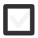# What is PRIME NUMBERS? Which are the first 5? Why is the binary number 1110 = 14?+1 vote
151 views
In 10-12
edited

## 1 lesson

by Guru (6.1k)
edited

Prime numbers

Prime numbers are numbers that are divisible only by themselves and 1.

Let’s learn the theory

Exercises:

Here are online worksheets about prime numbers

Mathscore Prime%20Numbers

Mount Royal University->Higher Arithmetic->Prime_numbers